# JP4745153B2 - Transmission power control apparatus and transmission power control method - Google Patents

## Info

Publication number
JP4745153B2
JP4745153B2 JP2006182540A JP2006182540A JP4745153B2 JP 4745153 B2 JP4745153 B2 JP 4745153B2 JP 2006182540 A JP2006182540 A JP 2006182540A JP 2006182540 A JP2006182540 A JP 2006182540A JP 4745153 B2 JP4745153 B2 JP 4745153B2
Authority
JP
Japan
Prior art keywords
transmission power
dpcch
power value
value
reception
Prior art date
Legal status (The legal status is an assumption and is not a legal conclusion. Google has not performed a legal analysis and makes no representation as to the accuracy of the status listed.)
Active
Application number
JP2006182540A
Other languages
Japanese (ja)
Other versions
JP2008011464A (en
Inventor

Original Assignee

Priority date (The priority date is an assumption and is not a legal conclusion. Google has not performed a legal analysis and makes no representation as to the accuracy of the date listed.)
Filing date
Publication date
Application filed by 富士通株式会社 filed Critical 富士通株式会社
Priority to JP2006182540A priority Critical patent/JP4745153B2/en
Publication of JP2008011464A publication Critical patent/JP2008011464A/en
Application granted granted Critical
Publication of JP4745153B2 publication Critical patent/JP4745153B2/en
Application status is Active legal-status Critical
Anticipated expiration legal-status Critical

## Images

•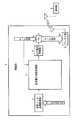•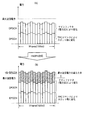•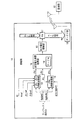••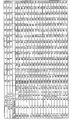•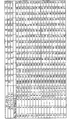•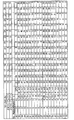•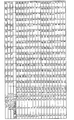•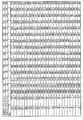•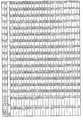•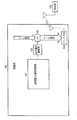•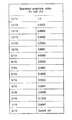•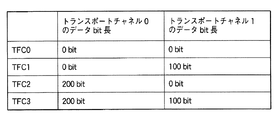•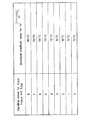•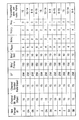•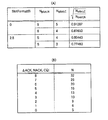•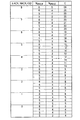•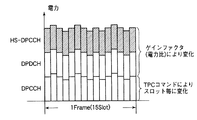• Y02B60/50

## Description

The present invention relates to a transmission power control apparatus and a transmission power control method for reducing a calculation processing amount of a transmission power value in a mobile station of a mobile communication system.

A mobile station in a mobile communication system is configured to control a transmission power amplifier by obtaining a necessary minimum transmission power value corresponding to transmission data, and to control so as not to exceed a prescribed maximum transmission power value It has. In addition, in order to check the receivable status with a base station other than the currently communicating base station at the time of handover, a compressed mode for forming a reception gap is applied, and the timing of the formed gap is applied. In this case, means for detecting the reception radio wave state and determining whether or not handover is appropriate is applied. In addition, HSDPA (High Speed Downlink Packet Access) that can transmit a large amount of data from a base station to a mobile station has been proposed.

This HSDPA is a system that transmits a large amount of data on the downlink from the base station to the mobile station separately from the normal channel, and the mobile station can receive an ACK if it can be normally received on the downlink. If not, a NACK is transmitted to each base station. Also, the quality of the downlink propagation state is converted into a value called CQI (Channel Quality Indicator) and transmitted from the mobile station to the base station. The ACK / NACK and the CQI are transmitted from the mobile station to the base station through a physical channel called HS-DPCCH (Dedicated Physical Control for HS-DSCH) (HS-DSCH; High Speed Downlink Shared Channel). During normal channel transmission / reception, control data is transmitted from the mobile station to the base station using a physical channel DPCCH (Dedicated Physical Control Channel) and user data is transmitted using the physical channel DPDCH (Dedicated Physical Data Channel). However, when HSDPA is applied, HS-DPCCH (control channel) is multiplexed together with DPCCH (control channel) and DPDCH (data channel) and transmitted to the base station.

FIG. 11 shows a main part of a conventional transmission power control function of a mobile station, where 100 is a mobile station, 101 is a transmission power calculation processing unit, 102 is an output voltage control unit, 103 is a transmission power amplifier (PA), and 104 is An antenna unit 105 indicates a base station. The transmission data is amplified by the transmission power amplifier 103 and transmitted to the base station 105 via the antenna unit 104. In this case, when the transmission power calculation processing unit 101 exceeds a specified maximum transmission power value, the transmission power calculation processing unit 101 performs processing to reduce the excess from the transmission power value of the DPCCH.

Further, between the mobile station 100 and the base station 105, the power (Pc) of the DPCCH determines whether or not the transmission from the mobile station 100 to the base station 105 is normal. Closed-loop power control is applied to the station 100 in which a 1 dB increase or decrease is instructed by TPC (Transmit Power Control) every slot. For example, in the case where transmission is performed from the mobile station 100 to the base station 105 at a level lower than the reference value generated by the base station 105, if the transmission is not normally received, the TPC gives an instruction to “up 1 dB” On the contrary, when the transmission is performed from the mobile station 100 to the base station 105 at a level higher than the reference value, when the transmission is normally received, the instruction “decrease 1 dB” is given by the TPC described above. Note that, when a call is connected between the mobile station 100 and the base station 105, an initial value of the DPCCH is designated, and from the initial value, in accordance with an instruction from the base station 105 for each slot, in ± 1 dB steps as described above Control to change is performed.

The transmission power of data transmitted from mobile station 100 to base station 105 is the sum of the powers of DPCCH and DPDCH in the normal mode. The power of DPDCH can be calculated from gain factors βc and βd which are amplitude ratios of DPCCH and DPDCH in the normal mode, and can be calculated from gain factors βc and βd and DPCCH transmission power Pc. Similarly, in the presto mode, the transmission power can be calculated from Pc, βd, and βc. However, as described above, the gap is formed between the frames, so that βd and βc are calculated in the normal mode (non-conversion mode). (Presto mode).

In addition, since HS-DPCCH is added during HSDPA, βhs, which is the amplitude ratio of HS-DPCCH, is calculated in addition to βc, βd, which are amplitude ratios of DPCCH and DPDCH. The transmission power at the time of HSDPA is the sum of transmission power corresponding to DPCCH, DPDCH, and HS-DPCCH. The transmission power of HS-DPCCH can be calculated from βc, βd, βhs and Pc.

The ratio (Aj) of the gain factor between DPCCH and DPDCH in the non-compressed mode can be obtained from (Equation 1).
Aj = βd, ref / βc, ref · √ (Kj / Kref) (Equation 1)
βd, ref: DPDCH gain factor of reference (notified from higher layer)
βc, ref: DPCCH gain factor of reference (notified from higher layer)
Kref: Kref = Σ i RM i · Nref i
RM i : Rate matching rate of reference TFC (notified from higher layer)
Nref i : Data bit length of reference TFC Kj: Kj = Σ i RM i · N i
RM i : TFC rate matching rate (notified by higher layer)
N i : Data bit length of TFC i: Transport channel number √ (Kj / Kref) indicates the square root of (Kj / Kref).
Further, which TFC is the reference TFC is notified from the upper layer. A combination of transport channels is referred to as the above-mentioned TFC (Transport Format Combination).

The gain factor of DPCCH with respect to TFC is βc, the gain factor of DPDCH is βd, and βd and βc are calculated as follows. With respect to the gain factor ratio Aj, when Aj> 1, βd = 1.0, and βc is the maximum value within 1 / Aj in the quantized amplitude ratio shown in FIG. Applicable (however, if βc is less than 1/15, βc = 1/15). When Aj ≦ 1, βd is set to a minimum value equal to or larger than Aj in FIG. 12, and βc = 1.0. As a specific example, βd, ref: 15, βc, ref: 12, and an example of each TFC and the data bit length of the transport channel are shown in FIG.

In this case, when the rate matching rate of transport channel 0: RM0 = 3, the rate matching rate of transport channel 1: RM1 = 1, and TFC3 is the reference TFC, the gain factor of TFC2 is as shown below. . The data bit length of transport channel 0 of TFC3 is 200 bits, the data length of transport channel 1 is 100 bits, the data bit length of transport channel 0 of TFC2 is 200 bits, and the data bits of transport channel 1 Since the length is 0 bits,
Kref = (3 × 200) + (1 × 100) = 700
Kj = (3 × 200) + (1 × 0) = 600
Aj = (15/12) × √ (600/700) = 1.1572
Since Aj> 1, βd = 1, and 1 / Aj = 0.8641, βc = 12/15.
It becomes. From the above, βd: βc = 15: 12. That is, the amplitude ratio between DPDCH and DPCCH of TFC2 is 15:12.

Further, the gain factor βhs of HS-DPCCH is set by calculating ACK / NACK / CQI from βc. The formula for calculating the gain factor βhs in the non-compressed mode is shown below.
βhs = βc × 10 ^ (ΔHS -DPCCH / 20) (Expression 2)
10 ^ (ΔHS -DPCCH / 20): Indicates 10th power of (ΔHS -DPCCH / 20).
βc: Gain factor given to DPCCH Δ HS-DPCCH : Used to calculate the power offset between DPCCH and HS-DPCCH. When an HS-CH call is connected, the ACK / NACK / CQI values of ΔACK, ΔNACK, ΔCQI ( (Values of 0 to 8) are notified, and the HS-DPCCH transmission state at that time is as follows.
When ACK is sent: Δ HS-DPCCH = ΔACK
During NACK transmission: ΔHS -DPCCH = ΔNACK
During CQI transmission: Δ HS-DPCCH = ΔCQI

FIG. 14 shows a power offset (amplitude magnitude with respect to DPCCH) with respect to the gain factor βc using the aforementioned ΔHS-DPCCH . Since HS-DPCCH is the number of uplink DPDCHs = 1 (odd number), it is code-multiplexed on DPCCH (Q-ch). In addition, for a slot in which HS-DPCCH (ACK, NACK, CQI) is code-multiplexed, a βhs value is multiplied as the amplitude ratio of HS-DPCCH.

The ratio (As, j) of the gain factor of DPCCH and DPDCH in the compressed mode can be obtained by (Equation 3).
Ac, j = Aj · √ (15 · N pilot, C / N slots, C · N pitot, N ) ·· (Equation 3)
N pilot, C : number of pilot bits per slot in compressed frame N pilot, N : number of pilot bits per slot in normal time (non-compressed frame) N slots, C : transmission slot in compressed frame Number Aj: Normal gain factor ratio

When the gain factor of DPCCH with respect to TFCI of a compressed frame is β c, C, j and the gain factor of DPDCH is β d, C, j , β c, C, j · β d, C, j are as follows: Is calculated as follows.
When Ac, j> 1: β d, C, j = 1.0, and β c, C, j applies the maximum value in FIG. 12 that is 1 / Ac, j or less. (However, if the beta c, C, j is less than 1/15, beta c, C, and j = 1/15.)
When Ac, j ≦ 1: β d, C, j is the minimum value in FIG. 12 that is equal to or greater than AC, j, and β c, C, j = 1.0.

The calculation of the gain factor β hs, C of the compressed frame can be performed by the following (Equation 4).
β hs, C = β c, C × 10 ^ (ΔHS -DPCCH / 20) × √ (N pilot, C / N pilot, N )
... (Formula 4)
β c, C: the compressed frame beta c
Δ HS-DPCCH : N pilot as in normal mode (non-compressed) , C : Number of pilot bits per DPCCH slot in compressed frame N pilot, N : Number of pilot bits per DPCCH slot in non-compressed frame

FIG. 15 is an explanatory diagram of the slot format of the DPCCH. The slot format (Slot Format), the channel bit rate (Channel Bit Rate (kbps)), the channel symbol rate (Channel Symbol Rate (kssps)), SF, and The number of bits per frame (Bits / Frame), the number of bits per slot (Bits / Slot), the number of bits N pilot , N TPC , N TFCI , N FB , the number of slots per transmission frame, etc. Yes. The pilot bit number N pilot, C can be obtained from N pilot by the slot format of DPCCH and * A, * B. Also, the DPCCH slot format is notified from the host when an individual CH call is connected. * A and * B can be determined from the number of transmission slots per frame of a compressed frame (a frame with a gap). That is, * A: 10 slots / frame or more, * B: less than 10 slots / frame, and the compressed gap is a maximum of 7 slots / frame.

There are six combinations of N pilot, C and N pilot, N , two for slot formats 0, 2, and 5, respectively , and only combinations of N pilot, C and N pilot, N. Then, there are four ways as shown in FIG. When β hs of the non-compressed frame is obtained, it is obtained by the following (formula 5).
βhs = βc × 10 ^ (ΔHS -DPCCH / 20) = βc × N (Expression 5)
Incidentally, N of at beta c × N in (Equation 5), due to rounding, can be used ΔACK shown in (B) of FIG. 16, DerutaNACK, nine kinds of values corresponding to the value of DerutaCQI. Then, multiplication with βc (1 to 15) is performed in each frame for ACK, NACK, and CQI to obtain β hs_ack , β hs_nack , and β hs_cqi .

In the compressed mode, the gain factor β hs is obtained by (Expression 6) obtained by modifying (Expression 4).
β hs, C = β c, C × 10 ^ (ΔHS -DPCCH / 20) × √ (N pilot, C / N pilot, N ) = β c, C × C (Formula 6)
It should be noted that the values of 4 to 29 shown in FIG. 17 can be used as C in βc and C × C in (Expression 6). In order to calculate the gain factor βhs, values shown in FIG. 17 are used corresponding to the values of ΔACK, ΔNACK, ΔCQI and the values of N pilot, C , N pilot, N. In each frame, multiplication with βc (1 to 15) is performed for each of ACK, NACK, and CQI to obtain β hs_ack , β hs_nack , and β hs_cqi .

When HSDPA is supported, there are three types of data to be transmitted: HS-DPCCH in addition to DPCCH and DPDCH. Among these, the DPCCH changes according to the TPC command for each slot, while the DPDCH and HS-DPCCH change depending on the power ratio (gain factor). When a mobile station transmits, it is amplified by a transmission power amplifier (PA), and the signal level input to this transmission power amplifier (PA) and the signal level actually output from the transmission power amplifier (PA) It is known that if the value exceeds a certain value, it does not become a proportional transmission power value due to the nonlinear characteristic of the transmission power amplifier (PA). If an excessive level of transmission data is input to the transmission power amplifier (PA), there is a high possibility that the transmission system including the transmission power amplifier (PA) will be damaged. May interfere with the channel. Therefore, the transmission power amplifier (PA) is controlled so as not to exceed the maximum transmission power.

In HSDPA, for the HS-DSCCH received by the mobile station, ACK is transmitted in the case of CRC OK, and NACK is transmitted in the case of CRC NG. Also, the quality of data received by the mobile station is periodically transmitted to the base station by CQI (Channel Quality Indicator). This ACK / NACK and CQI are transmitted as HS-DPCCH. In terms of power value, HS-DPCCH is added to conventional DPCCH + DPDCH.

The transmission power value is calculated by the transmission power calculation processing unit so that the transmission power value does not exceed the maximum transmission power value, and the transmission power amplifier (PA) is controlled from the output voltage control unit according to the calculated transmission power value. The calculation processing method is
When transmission power value (DPCCH transmission power value + DPDCH transmission power value)> maximum transmission power value,
Δ = transmission power value (DPCCH transmission power value + DPDCH transmission power value) −maximum transmission power value is calculated,
DPCCH transmission power value = DPCCH transmission power value−Δ
, And the transmission power amplifier (PA) is controlled by reducing the amount (Δ) that exceeds the transmission power value on the DPCCH side.

In HSDPA, when the same transmission power amplifier (PA) is used, the situation where the transmission power value exceeds the maximum transmission power value occurs more frequently than the conventional example in which HSDPA is not applied. In this case, the power of DPCCH The value will be reduced. When the power value of the DPCCH is reduced, there is a high probability that information such as TPC commands and TFCI transmitted from the mobile station to the base station will be lost, closed loop power control failure, transmission data from the mobile station to the base station There is a high possibility that communication of uplink signals from the mobile station to the base station will be interrupted.

Also, the downlink DPCCH data quality in the direction from the base station to the mobile station is monitored by a TPC command (command for instructing to increase / decrease the transmission power from now on), and if the quality is lower than a certain level, the downlink quality is poor. Notification is made to increase the power, and conversely if the quality is better than a certain level, the quality of the downlink is too good, and notification is made to lower the transmission power. From the mobile station to the base station, a TPC bit is inserted in the DPCCH and transmitted. TFCI is an indicator indicating TFC, and is transmitted from the mobile station to the base station by inserting the TFCI bit into the DPCCH. The base station analyzes the TFCI and recognizes the DFC (User Data Channel) TFC transmitted simultaneously. As described above, the conventional mobile station performs communication with the base station.

Further, in the mobile station in the conventional mobile communication system to which the W-CDMA system is applied, the threshold transmission power of the control channel that maximizes the transmission power is set as the gain for level control of the data channel and the control channel. A means for providing a control channel threshold power table based on the combination of factors and the maximum transmission power and performing a transmission process according to the maximum transmission power restriction has been proposed (for example, see Patent Document 1).
JP 2006-20234 A

In the conventional mobile station, as described with reference to FIG. 11, the transmission power calculation processing unit 101 performs transmission power calculation processing so as not to exceed the maximum transmission power value when calculating the transmission power value. Based on the transmission power value calculated by unit 101, output voltage control unit 102 controls transmission power amplifier 103 to amplify transmission data and transmit it from antenna unit 104 to base station 105. The transmission power in a configuration that does not support HSDPA is the sum of the power values of DPCCH and DPDCH in normal mode and compressed mode, but when HSDPA is supported, the transmission power of DPCCH and DPDCH The power value of HS-DPCCH is added to the sum of the power values. In addition, since the power value of the DPCCH changes for each slot, it is necessary to calculate the total power value for each slot.

When HSDPA is supported, the HS-DPCCH power value may be added to exceed the preset maximum transmission power value. In this case, the DPCCH transmission power value is reduced by the amount exceeding the maximum transmission power value so that the entire transmission power value does not exceed the maximum transmission power value. Therefore, as described above, there is a high probability that information such as TPC commands and TFCI transmitted from the mobile station to the base station is lost, the closed loop power control is broken, and the format of transmission data from the mobile station to the base station is increased. Misrecognition, etc. occur, and there is a high possibility that communication of uplink signals from the mobile station to the base station will be interrupted, making it impossible to continue high-speed data reception by HSDPA.

In addition, a transmission power correction value is required to calculate the transmission power value. In the case of the conventional method (not compatible with HSDPA), only the gain factors βc and βd have an effect on the calculation of the transmission power correction value. Therefore, it was possible to perform calculation only with a combination of βc and βd. However, in the case of HSDPA compatibility, there is a problem that the calculation process of the transmission power correction value becomes complicated due to the addition of the gain factor βhs. For example, as shown in FIG. 18, when one frame is 15 slots, and DPCCH, DPDCH, and HS-DPCCH change for each slot and become a value exceeding the maximum transmission power value, conventionally, as described above, Then, control for subtracting the amount exceeding the maximum transmission power value uniformly from the transmission power value of the DPCCH was performed. However, there is a problem that the error of DPCCH increases.

In addition, the above-mentioned Patent Document 1 is provided with a control channel threshold power table to shorten the processing time for transmission power control. There was a problem that was not considered.

The present invention solves the conventional problems, and performs transmission power control corresponding to reception quality and simplifies arithmetic processing.

The transmission power control apparatus according to the present invention is a transmission power control apparatus that calculates a transmission power value from a mobile station to a base station and controls a transmission power amplifier, and that detects reception quality with the base station. A data quality monitoring unit, a transmission power value superimposed on a data channel to be transmitted to the base station and a single or a plurality of types of control channels, and the transmission power value exceeding a preset maximum transmission power value A transmission power calculation processing unit for reducing transmission power values for the control channels set in advance of the plurality of types of control channels in correspondence with the reception quality information from the reception data quality monitoring unit.

Further, in a transmission power control apparatus that calculates a transmission power value from a mobile station to a base station and controls a transmission power amplifier, the received data by the high-speed downlink packet access (HSDPA) from the base station A reception data quality monitoring unit for detecting reception quality, a data channel (DPDCH) to be transmitted to the base station, a control channel (DPCCH), and a control channel (HS-DPCCH) by the high-speed downlink packet access (HSDPA) ) And the transmission power value exceeding the preset maximum transmission power value, the reception quality information from the reception data quality monitoring unit indicates a reception state where the reception quality information is equal to or greater than a predetermined value. When the transmission quality value is reduced from the transmission power value of the control channel and the reception quality information indicates a reception state of a predetermined value or less, And a transmission power calculating section for reducing the transmission power value of the control channel by High Speed Downlink Packet Access (HSDPA).

Also, in a transmission power control apparatus that controls a transmission power amplifier by calculating a transmission power value from a mobile station to a base station, to calculate the transmission power value from a transmission power value of a control channel and a transmission power correction value. The transmission power correction value of the normal mode, the transmission power correction value table based on the combination of the gain factors of the data channel and the control channel, and the compressed mode based on the combination of the gain factors of the data channel and the control channel for the compressed mode. Transmission power correction value table and the transmission power correction value from the normal mode transmission power correction value table or the compressed mode transmission power correction value table are added to the transmission power value of the control channel to obtain the total transmission power value. A transmission power calculation processing unit including a calculation means to be obtained.

The transmission power correction value table in the compressed mode of the transmission power calculation processing unit corresponds to the ratio between the number of pilot bits of the compressed frame per slot of the control channel and the number of pilot bits per slot of the non-compressed frame. The transmission power correction value in the compressed mode based on the combination of the obtained coefficient and the gain factor of the compressed mode data channel and the control channel is stored.

The transmission power control method of the present invention is a transmission power control method for controlling a transmission power amplifier by calculating a transmission power value from a mobile station to a base station. The transmission power value detected by the quality monitoring unit and superimposed on the data channel to be transmitted to the base station and the single or plural types of control channels is calculated, and the transmission power value exceeds the preset maximum transmission power value. The transmission power calculation process for reducing the transmission power value for the preset control channel of the plurality of types of control channels corresponding to the reception quality information from the reception data quality monitoring unit.

Also, in a transmission power control method for calculating a transmission power value from a mobile station to a base station and controlling a transmission power amplifier, the received data by the high speed downlink packet access (HSDPA) from the base station The reception quality is detected by the reception data quality monitoring unit, and the transmission power value is calculated by superimposing the data channel to be transmitted to the base station, the control channel, and the control channel by the high-speed downlink packet access (HSDPA). When the reception quality information from the reception data quality monitoring unit indicates a reception state equal to or greater than a predetermined value, the transmission power value of the control channel indicates that the transmission power value exceeds a preset maximum transmission power value. When the reception quality information indicates a reception state below a predetermined value, the high-speed downlink packet access It is intended to include the transmit power calculation process to reduce the transmission power value of the control channel by (HSDPA).

When the transmission power value calculated by the transmission power calculation processing unit exceeds the maximum transmission power value, based on the reception quality information, the transmission power of one of a plurality of types of control channels, that is, DPCCH and HS-DPCCH The control channel HS-DPCCH is processed so that HSDPA can be continued when the reception quality is maintained at a good reception quality equal to or higher than a predetermined value. If the DPCCH transmission power value is reduced and the reception quality is below a predetermined value and indicates a bad reception state, it is difficult to continue HSDPA, so the DPCCH transmission power value remains unchanged. By reducing the transmission power value of HS-DPCCH, it is possible to control the calculation processing of the optimum transmission power value corresponding to the reception quality information.

In addition, by providing a table storing transmission power correction values necessary for calculating the transmission power value in the transmission power calculation processing unit, the calculation processing load is reduced and the calculation of the transmission power value corresponding to the slot becomes easy. .

The transmission power control apparatus according to the present invention will be described with reference to FIG. 1. A reception data quality monitoring unit 7 that detects reception quality of reception data from the base station 2 by high speed downlink packet access (HSDPA). And a transmission power value in which a data channel (DPDCH) to be transmitted to the base station 2, a control channel (DPCCH), and a control channel (HS-DPCCH) by high speed downlink packet access (HSDPA) are superimposed. When the reception quality information from the reception data quality monitoring unit 7 indicates a reception state of a predetermined value or more, the amount of transmission power value exceeding the preset maximum transmission power value indicates transmission of the control channel (DPCCH). When it is reduced from the power value and the reception quality information indicates a reception state below the specified value, the high-speed downlink packet access And a transmission power calculating unit 3 to reduce the transmission power value of control channel (HS-DPCCH) by (HSDPA).

In the transmission power control method of the present invention, the reception data quality monitoring unit 7 detects the reception quality of the reception data from the base station 2 by the high speed downlink packet access (HSDPA), and the data to be transmitted to the base station 2 The transmission power value is calculated by superimposing the control channel (HS-DPCCH) by the channel (DPDCH), the control channel (DPCCH), and the high-speed downlink packet access (HSDPA), and this transmission power value is set in advance. When the reception quality information from the reception data quality monitoring unit 7 indicates a reception state that exceeds a predetermined value, the amount exceeding the maximum transmission power value is reduced from the transmission power value of the control channel (DPCCH), and the reception quality information When the reception status is below the specified value, it is controlled by high speed downlink packet access (HSDPA). It is intended to include the transmit power calculation process to reduce the transmission power value of channel (HS-DPCCH).

FIG. 1 is an explanatory diagram of a main part of the first embodiment of the present invention, in which 1 is a mobile station, 2 is a base station, 3 is a transmission power calculation processing unit, 4 is an output voltage control unit, and 5 is a transmission power amplifier (PA). ), 6 indicates an antenna unit, and 7 indicates a received data quality monitoring unit. Although the reception processing means is not shown, various known reception processing means can be applied. Also, the transmission data processing means in the preceding stage of the transmission power amplifier 5 is not shown, but various known means can be applied.

The transmission data is amplified by the transmission power amplifier 5 and transmitted to the base station 2 via the antenna unit 6. The transmission power calculation processing unit 3 obtains a transmission power value corresponding to DPCCH, DPDCH, and HS-DPCCH to be transmitted to the base station 2 and obtains a transmission power value that does not exceed the maximum transmission power value. Input to the control unit 4, the amplification factor of the transmission power amplifier 5 is controlled from the output voltage control unit 4, the transmission data is amplified by the transmission power amplifier 5, and transmitted to the base station 2 through the antenna unit 6.

The reception data quality monitoring unit 7 shows a case where reception quality is monitored based on HS-DSCH data received and processed by a reception processing means (not shown). For example, HS-DSCH block error rate (BLER) monitoring The result is input to the transmission power calculation processing unit 3 as reception quality information. The transmission power calculation processing unit 3 calculates a transmission power value for transmitting DPCCH, DPDCH, and HS-DSCH corresponding to a data channel and a plurality of types of control channels to the base station 2 according to the reception quality information.

For example, in the HS-DSCH from the base station, one transport block exists in 2 ms, and a CRC bit (24 bits) is assigned to one transport block. The mobile station 1 can calculate the block error rate BLER as follows according to the ratio of the total number of transport blocks of HS-DSCH received within a certain period of time and the number of CRC NG among them. it can.
BLER = number of CRC NG / total number of HS-DSCH transport blocks The received data quality monitoring unit 7 calculates a block error rate BLER from the received HS-DSCH data, and sends it to the transmission power calculation processing unit 3 as reception quality information. input. When the block error rate BLER is smaller than the predetermined value, the transmission power calculation processing unit 3 can determine that the downlink HS-DSCH reception state is good. On the other hand, when the block error rate BLER is larger than a predetermined value, it can be determined that the reception state of the downlink HS-DSCH is bad.

HS-DSCH block error rate BLER is 0.1 (10% error rate) in a static environment and 0.2 to 0.3 (20% to 30% error rate) in a normal environment For example, when an error rate exceeding 50% can be observed, it can be determined that the reception quality of HS-DSCH is very poor. HSDPA can receive a large amount of data, but the reception quality of HS-DSCH deteriorates, and if the network side determines that it cannot receive a large amount of data, it transitions to the conventional data exchange on DPCH. , The HSDPA call is disconnected, and communication of downlink HS-DSCH / uplink HS-DPCCH data is lost.

Therefore, the transmission power calculation processing unit 3 determines that the HSDPA call is just before disconnection based on the reception quality information indicating that the reception quality of the HS-DSCH has deteriorated, and determines that the DPCCH is more important than the HS-DPCCH. However, when the transmission power value becomes larger than the maximum transmission power value, the transmission power value of HS-DPCCH instead of DPCCH is reduced. When the reception quality information indicates that the reception state of the HS-DSCH is good, it is determined that the HSDPA call is continued, and the transmission power value is clipped so as to reduce the transmission power of the DPCCH.

For example, as shown in FIG. 2A, when one frame has 15 slots and is not compatible with HSDPA, DPDCH is changed by a gain factor and DPCCH is changed by a TPC command, and transmitted without exceeding the maximum transmission power value. In the case of being controlled, HS-DPCCH is added and the calculated transmission power value may exceed the preset maximum transmission power value as shown in FIG. Become more. Conventionally, when the maximum transmission power value is exceeded, the transmission power value of the DPCCH is controlled so as not to exceed the maximum transmission power value. However, in the present invention, as described above, reception is performed. According to the quality information, a plurality of types of control channels, that is, DPCCH or HS-DPCCH are selected, and transmission power value clipping processing is performed. Thereby, when the reception state of the HS-DSCH is deteriorated, it is possible to continue normal transmission / reception excluding at least HSDPA.

FIG. 3 is an explanatory diagram of the main part of the second embodiment of the present invention, in which 11 is a mobile station, 12 is a base station, 13 is a βc, βd calculation processing unit, 14 is a βhs calculation processing unit, and 15 is a transmission power calculation process. , 16 is an output voltage control unit, 17 is a power amplifier (PA), 18 is an antenna unit, and 19 is a table. As in the case shown in FIG. 1, the reception processing means is not shown, but various known reception processing means can be applied. Although the transmission data processing means in the previous stage of the transmission power calculation processing unit 15 is not shown, various known means can be applied. The βc, βd arithmetic processing unit 13 also includes the above-described Kj, Kref, βc, ref, βd, ref and the number of pilot bits Npilot C, NpilotN per slot in the compressed mode (usually, “N pilot, C , N pilot, N ″) are input, gain factors βc and βd are calculated , and input to the transmission power calculation processing unit 15. The βhs arithmetic processing unit 14 includes Δhs-dpoch (usually expressed as “ΔHS -DPCCH ”), NpilotC and NpilotN (usually “N pilot, C ” and “N pilot, N ″) and βc from the βc, βd arithmetic processing unit 13 are input, and the calculated βhs is input to the transmission power arithmetic processing unit 15. The table 19 stores transmission power correction values formed based on the gain factors βd, βc, βhs, and reduces the processing amount of transmission power calculation.

HS-DPCCH is added to DPCCH and DPDCH, and the total power P when HSDPA is supported is the total power (P) and each power (Pc, Pd, Phs) of DPCCH, DPDCH, and HS-DPCCH. The relationship is as follows.
P = Pc + Pd + Phs (Expression 8)
The DPCCH power Pc varies from slot to slot, the DPDCH power Pd is determined by Pc and gain factors βd and βc, and the HS-DPCCH power Phs is determined by Pc and gain factors βhs and βc.

Accordingly, the relationship between each transmission power value and the gain factor is as follows (note that the power ratio is the square of the amplitude ratio).
Pc: Pd = βc 2 : βd 2 (Formula 7-1)
Pc: Phs = βc 2 : βhs 2 (Formula 7-2)
Based on these formulas, the power Pd of DPDCH and the power Phs of HS-DPCCH are as shown in the following formula.
Pd = Pc × (βd 2 / βc 2 ) (Formula 9)
Phs = Pc × (βhs 2 / βc 2 ) (Formula 10)

The transmission power P when HS-DPCCH is supported is
P = Pc + Pc × (βd 2 / βc 2 ) + Pc × (βhs 2 / βc 2 )
= Pc × (βd 2 + βc 2 + βhs 2 ) / βc 2 (Formula 11)
And if you do the decibel notation,
P = Pc + 10 log 10 {(βd 2 + βc 2 + βhs 2 ) / βc 2 }
= Pc + 20log 10 (√ (βd 2 + βc 2 + βhs 2 ) / βc 2 ) (Formula 12)
It becomes. That is, it can be seen that the transmission power P is obtained by adding DPCCH power Pc (dBm) and DPDCH + HS-DPCCH power 20 log 10 (√ (βd 2 + βc 2 + βhs 2 ) / βc 2 ) (dBm). Note that √ (βd 2 + βc 2 + βhs 2 ) represents a square root in parentheses.

As described above, the pattern of the gain factor βhs in the compressed mode compatible with HSDPA is 540 patterns in total when βc: 15, N pilot, N , Npott, C : 4, and ΔHS -DPCCH : 9. . Therefore, based on (Equation 12), the transmission power P is obtained using the transmission power correction value b as follows. That is,
P = Pc + b
b = 20 log 10 (√ (βd 2 + βc 2 + βhs 2 ) / βc 2 ) (Formula 13)
Represent as

The transmission power correction value b can be obtained from the gain factors βd, βc, βhs, and is stored in the table 19 in advance corresponding to the combination. In this case, it can be classified into two types for the normal frame and for the compressed frame, depending on whether or not the compressed mode is applied. Compared to floating point arithmetic, fixed point arithmetic generally has a shorter arithmetic processing time, and in order to improve the accuracy of arithmetic processing using the transmission power correction value b, for example, eight times. When considering accuracy, (Expression 13) is expressed by the following expression: b = {8 × 20 log 10 (√ (βd 2 + βc 2 + βhs 2 ) / βc 2 )} (Expression 14)

When there is no HS-DPCCH, the table shown in FIG. 4 can be formed by a combination of gain factors βd (0 to 15) and βc (1 to 15). In addition to DTXb (transmission power correction value without HS-DPCCH) for each frame, a transmission power correction value b (Δ8 to Δ0) is obtained from the table by a combination of gain factors βd and βc for each of ACK, NACK, and CQI. be able to.

For compressed frames, in addition to gain factors βc, c (compressed βc) and βd, c (compressed βd), table contents are added for gain factors βhs, c (compressed βhs). It becomes. The patterns of the gain factors βhs, c are βc, c, ΔACK, ΔNACK, ΔCQI (Δ8-0) and NpilotC, NpilotN (= the number of pilot bits per DPCCH slot of the above-mentioned non-compressed frame, N pilot, N and compressed It varies depending on the value of the number of pilot bits per pilot DPCCH slot (N pilot, C ). Therefore, the table of transmission power correction values at the time of HSDPA compatible compressed frames is determined by a combination of βd, c, βc, c, ΔACK, NACK, CQI (Δ8 to 0), NpilotN, and NpilotC. Among them, there are 30 patterns of βd, c, βc, c, and there are 36 (9 × 4) patterns of ΔACK, ΔNACK, ΔCQI (Δ8-0), NpilotN, and NpilotC, which are shown in FIGS. By providing the table 19 of contents in the transmission power calculation processing unit 15, the transmission power correction value b can be obtained with reference to this table 19, and the amount of calculation processing can be reduced.

The transmission power correction value b in the compressed frame is a combination of gain factors βd, c, βc, c for each of ACK, NACK, and CQI, and ΔACK, ΔNACK, ΔCQI (Δ8 to Δ0), NpilotN, which determines βhs, c. By referring to the table according to the value of NpilotC, a total of four types of transmission power correction values can be obtained. The above-described tables shown in FIGS. 4 to 8 can be realized with a size of 300 + 1110 = 1410 (word).

The calculation of the total power P is performed for each slot, and further includes logarithmic processing, square root processing, and the like, so that the processing time becomes long. Further, in the case of HSDPA support, the transmission power is added by the amount of HS-DPCCH, so that the calculation process becomes more complicated. However, as described above, by storing the transmission power correction value b in the table 19 in advance, the time required for calculating the transmission power value can be shortened.

9 and 10 are explanatory diagrams of the table of the transmission power calculation processing unit according to the third embodiment of the present invention, and transmission in the compressed mode stored in the table 19 of the transmission power calculation processing unit 15 in FIG. It shows the contents of the power correction value. As shown in the above (formula 6), the gain factors β hs, C are represented by the product of β c, C and the coefficient C. Since β c, C (βc (compressed)) is the horizontal axis of the table, it is determined by the value of the coefficient C (see (Equation 6)), and the value of the coefficient C is 4 to 29. , ΔACK, ΔNACK, ΔCQI (Δ0 to Δ8), N pilot, N , N pilot, C even if the values of the coefficient C are duplicated, such duplication is included. The table configuration has a reduced number of parts. As a result, the configuration of 30 × 26 = 780 (words) can be realized.

The transmission power calculation processing unit 15 can quickly obtain the transmission power value in the compressed mode corresponding to HS-DPCCH without degrading the calculation accuracy by referring to such table contents.

It is principal part explanatory drawing of Example 1 of this invention. It is explanatory drawing of transmission power. It is principal part explanatory drawing of Example 2 of this invention. It is explanatory drawing of the transmission power correction value table at the time of the non-compressed mode of Example 2 of this invention. It is explanatory drawing of the transmission power correction value table at the time of the compressed mode of Example 2 of this invention. It is explanatory drawing of the transmission power correction value table at the time of the compressed mode of Example 2 of this invention. It is explanatory drawing of the transmission power correction value table at the time of the compressed mode of Example 2 of this invention. It is explanatory drawing of the transmission power correction value table at the time of the compressed mode of Example 2 of this invention. It is explanatory drawing of the transmission power correction value table at the time of the compressed mode of Example 3 of this invention. It is explanatory drawing of the transmission power correction value table at the time of the compressed mode of Example 3 of this invention. It is principal part explanatory drawing of the transmission power control function of a prior art example. It is explanatory drawing of a gain factor and a ratio. It is explanatory drawing of an example of a transport channel and a data bit length. It is explanatory drawing of a power offset. It is explanatory drawing of a DPCCH slot format. It is explanatory drawing of the value of N at the time of the combination of slot format at the time of a compressed mode, and a gain factor calculation. It is explanatory drawing of the value of C at the time of gain factor calculation. It is explanatory drawing of the transmission power at the time of compressed mode.

Explanation of symbols

DESCRIPTION OF SYMBOLS 1 Mobile station 2 Base station 3 Transmission power calculation processing part 4 Output voltage control part 5 Transmission power amplifier (PA)
6 Antenna unit 7 Received data quality monitoring unit

## Claims (2)

1. In a transmission power control apparatus for controlling a transmission power amplifier by calculating a transmission power value from a mobile station to a base station,
A reception data quality monitoring unit for detecting reception quality of reception data by high-speed downlink packet access from the base station;
A transmission power value is calculated by superimposing the data channel to be transmitted to the base station, the control channel, and the control channel by the high speed downlink packet access , and the transmission power value exceeds a preset maximum transmission power value. other content, when the reception quality information from the received data quality monitoring section indicates the reception state of the predetermined value or more, to reduce the transmission power value of the control channel, the reception state of the reception quality information is less than a predetermined value when showing a, the transmission power control apparatus characterized by comprising a transmission power calculating section for reducing the transmission power value of the control channel by the high-speed downlink packet access.
2. In a transmission power control method for controlling a transmission power amplifier by calculating a transmission power value from a mobile station to a base station,
A reception data quality monitoring unit detects reception quality of reception data by high-speed downlink packet access from the base station, and transmits to the base station a data channel, a control channel, and the high-speed downlink packet A transmission power value superimposed with a control channel by access is calculated, and the reception quality information from the reception data quality monitoring unit is equal to or greater than a predetermined value by the amount that the transmission power value exceeds a preset maximum transmission power value When the reception state is indicated, the transmission power value of the control channel is reduced, and when the reception quality information indicates a reception state below a predetermined value, the control channel is transmitted by the high speed downlink packet access. Includes transmit power calculation process to reduce power value
A transmission power control method characterized by the above.
JP2006182540A 2006-06-30 2006-06-30 Transmission power control apparatus and transmission power control method Active JP4745153B2 (en)

## Priority Applications (1)

Application Number Priority Date Filing Date Title
JP2006182540A JP4745153B2 (en) 2006-06-30 2006-06-30 Transmission power control apparatus and transmission power control method

## Applications Claiming Priority (1)

Application Number Priority Date Filing Date Title
JP2006182540A JP4745153B2 (en) 2006-06-30 2006-06-30 Transmission power control apparatus and transmission power control method

## Publications (2)

Publication Number Publication Date
JP2008011464A JP2008011464A (en) 2008-01-17
JP4745153B2 true JP4745153B2 (en) 2011-08-10

# Family

## Family Applications (1)

Application Number Title Priority Date Filing Date
JP2006182540A Active JP4745153B2 (en) 2006-06-30 2006-06-30 Transmission power control apparatus and transmission power control method

## Country Status (1)

JP (1) JP4745153B2 (en)

## Families Citing this family (1)

* Cited by examiner, † Cited by third party
Publication number Priority date Publication date Assignee Title
JP4787890B2 (en) 2009-04-21 2011-10-05 株式会社エヌ・ティ・ティ・ドコモ Communication terminal and method used in wireless communication system

## Citations (2)

* Cited by examiner, † Cited by third party
Publication number Priority date Publication date Assignee Title
WO2005076500A1 (en) * 2004-02-10 2005-08-18 Samsung Electronics Co., Ltd. An apparatus and a method for distributing a transmission power in a cellular communications network
JP2006014304A (en) * 2004-06-09 2006-01-12 Samsung Electronics Co Ltd Method and apparatus for data transmission in mobile communication system supporting enhanced uplink service

## Patent Citations (3)

* Cited by examiner, † Cited by third party
Publication number Priority date Publication date Assignee Title
WO2005076500A1 (en) * 2004-02-10 2005-08-18 Samsung Electronics Co., Ltd. An apparatus and a method for distributing a transmission power in a cellular communications network
JP2007520178A (en) * 2004-02-10 2007-07-19 サムスン エレクトロニクス カンパニー リミテッド Method and apparatus for allocating transmission power in a cellular communication network
JP2006014304A (en) * 2004-06-09 2006-01-12 Samsung Electronics Co Ltd Method and apparatus for data transmission in mobile communication system supporting enhanced uplink service

## Also Published As

Publication number Publication date
JP2008011464A (en) 2008-01-17

## Similar Documents

Publication Publication Date Title
CN1129250C (en) Device and method of continuous outer-loop power control in DTX mode for CDMA mobile communication system
JP5014820B2 (en) Mobile communication system, user apparatus and communication method
KR100923230B1 (en) Spread spectrum time division user equipment for multiple downlink time slots
US7657277B2 (en) Method and system for power control in a communication system
DE60034582T2 (en) Method for controlling the quality of service of a CDMA-based system
US6628924B1 (en) CDMA mobile communications system
EP2299760B1 (en) Mobile station and communication control method therefor
US7346034B2 (en) Cellular system, mobile station, base station and transmission power control method as well as program to be executed for implementing the method
EP1313232B1 (en) Method and apparatus for uplink transmission power control in a cdma communication system
ES2316371T3 (en) Adjustment of the sir threshold in a closed loop power control system.
US6832096B2 (en) Fast adaptive power control for a variable multirate communications system
US6173162B1 (en) Multiple code channel power control in a radio communication system
EP1216522B1 (en) Transmit power control
JP2011045106A (en) Method and apparatus for controlling transmission power while in soft handoff
RU2565030C2 (en) Device and method of controlling uplink power for wireless transmit/receive unit using multiple carriers
CN1196291C (en) Emitting power control method and mobile communication system
KR100429690B1 (en) Method and system for controlling the transmission power of certain parts of a radio transmission
AU2002220040B2 (en) Method and apparatus for power control in a wireless communication system
US7660600B2 (en) Adjustment of target signal-to-interference in outer loop power control for wireless communication systems
TWI364922B (en) Method of transmission power control of transmitting wireless transmit recieve unit(wtru) and recieving wtru
CN1243419C (en) Power control apparatus and method for W-CDMA communication system employing high-speed downlink packet access scheme
US7366130B2 (en) Base station and transmission power determining method in mobile communications systems
JP2010050999A (en) System and method to combine power control commands during soft handoff in ds/cdma cellular systems
EP1101311B1 (en) Transmitting and receiving device and method for continuous outer-loop power control while in dtx mode in a cdma mobile communication system
JP2004537228A (en) Method for determining whether to perform link adaptation in WCDMA communication

## Legal Events

Date Code Title Description
A621 Written request for application examination

Free format text: JAPANESE INTERMEDIATE CODE: A621

Effective date: 20090319

A977 Report on retrieval

Free format text: JAPANESE INTERMEDIATE CODE: A971007

Effective date: 20110124

A131 Notification of reasons for refusal

Free format text: JAPANESE INTERMEDIATE CODE: A131

Effective date: 20110208

A521 Written amendment

Free format text: JAPANESE INTERMEDIATE CODE: A523

Effective date: 20110408

TRDD Decision of grant or rejection written
A01 Written decision to grant a patent or to grant a registration (utility model)

Free format text: JAPANESE INTERMEDIATE CODE: A01

Effective date: 20110510

A01 Written decision to grant a patent or to grant a registration (utility model)

Free format text: JAPANESE INTERMEDIATE CODE: A01

A61 First payment of annual fees (during grant procedure)

Free format text: JAPANESE INTERMEDIATE CODE: A61

Effective date: 20110511

FPAY Renewal fee payment (event date is renewal date of database)

Free format text: PAYMENT UNTIL: 20140520

Year of fee payment: 3

R150 Certificate of patent or registration of utility model

Free format text: JAPANESE INTERMEDIATE CODE: R150